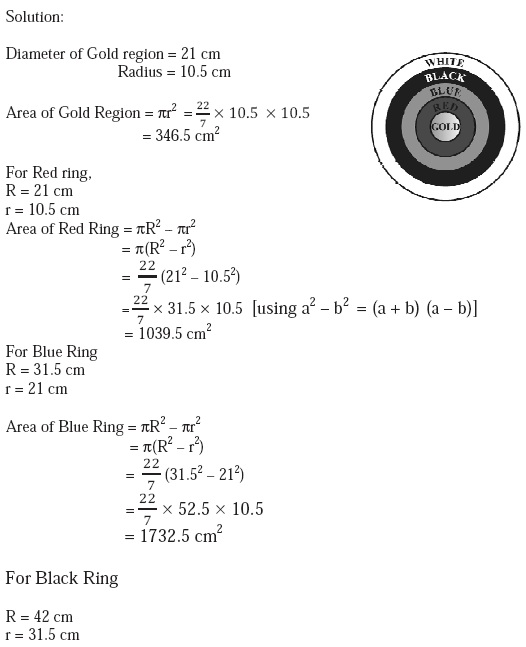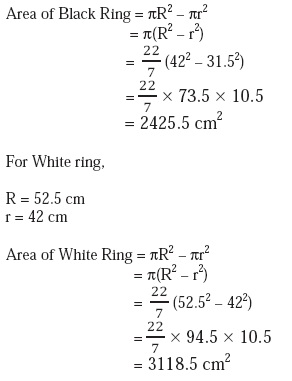Study Materials: ncert solutions

Our ncert solutions for Exercise 12.1 Class 10 maths 12. Areas Related to Circles - ncert solutions - Toppers Study is the best material for English Medium students cbse board and other state boards students.

Solutions ⇒ Class 10th ⇒ Mathematics ⇒ 12. Areas Related to Circles

# Exercise 12.1 Class 10 maths 12. Areas Related to Circles - ncert solutions - Toppers Study

Topper Study classes prepares ncert solutions on practical base problems and comes out with the best result that helps the students and teachers as well as tutors and so many ecademic coaching classes that they need in practical life. Our ncert solutions for Exercise 12.1 Class 10 maths 12. Areas Related to Circles - ncert solutions - Toppers Study is the best material for English Medium students cbse board and other state boards students.

## Exercise 12.1 Class 10 maths 12. Areas Related to Circles - ncert solutions - Toppers Study

CBSE board students who preparing for class 10 ncert solutions maths and Mathematics solved exercise chapter 12. Areas Related to Circles available and this helps in upcoming exams 2023-2024.

### You can Find Mathematics solution Class 10 Chapter 12. Areas Related to Circles

• All Chapter review quick revision notes for chapter 12. Areas Related to Circles Class 10
• NCERT Solutions And Textual questions Answers Class 10 Mathematics
• Extra NCERT Book questions Answers Class 10 Mathematics
• Importatnt key points with additional Assignment and questions bank solved.

NCERT Solutions do not only help you to cover your syllabus but also will give to textual support in exams 2023-2024 to complete exercise 12.1 maths class 10 chapter 12 in english medium. So revise and practice these all cbse study materials like class 10 maths chapter 12.1 in english ncert book. Also ensure to repractice all syllabus within time or before board exams for ncert class 10 maths ex 12.1 in english.

See all solutions for class 10 maths chapter 12 exercise 12 in english medium solved questions with answers.

### Exercise 12.1 class 10 Mathematics Chapter 12. Areas Related to Circles

• Exercise 12.1 Class 10 Maths 12. Areas Related To Circles - Ncert Solutions - Toppers Study
• Class 10 Ncert Solutions
• Solution Chapter 12. Areas Related To Circles Class 10
• Solutions Class 10
• Chapter 12. Areas Related To Circles Exercise 12.1 Class 10

## Exercise 12.1 Class 10 maths 12. Areas Related to Circles - ncert solutions - Toppers Study

### Exercise: 12.1

Q.1   The radii of two circles are 19 cm and 9 cm respectively. Find the radius of the circle which has circumference equal to the sum of the circumferences of the two circles.

Solution:

Let the radius of acquired circle = R

And radius of Large circle r1 = 19 cm

Radius of small circle r2 = 9 cm

Then,

2πR = 2πr1 + 2πr2

2πR = 2π (r1 + r2)

R = (r1 + r2)

= 19 + 9

= 28 cm

Q2.   The radii of two circles are 8 cm and 6 cm respectively. Find the radius of the circle having area equal to the sum of the areas of the two circles.

Solution:

Let the radius of acquired circle = R

And radius of Large circle r1 = 8 cm

Radius of small circle r2 = 6 cm

Then,

πR2 = πr12 + πr22

πR2 = π (r12 + r22)

R2 = (r12 + r22)

= 82 + 62

= 64 + 36 cm

= 100 cm

R = √100 = 10 cm

Q3.   Depicts an archery target marked with its five scoring areas from the centre outwards as Gold, Red, Blue, Black and White. The diameter of the region representing Gold score is 21 cm and each of the other bands is 10.5 cm wide. Find the area of each of the five scoring regions.Q4.  The wheels of a car are of diameter 80 cm each. How many complete revolutions does each wheel make in 10 minutes when the car is travelling at a speed of 66 km per hour?

Solution:Q5.   If the perimeter and the area of a circle are numerically equal, then find the radius of the circle.

Solution:

2πr = πr2

2r  = r2

2r  = r × r

r = 2 unit

##### Other Pages of this Chapter: 12. Areas Related to Circles

Important Study materials for classes 06, 07, 08,09,10, 11 and 12. Like CBSE Notes, Notes for Science, Notes for maths, Notes for Social Science, Notes for Accountancy, Notes for Economics, Notes for political Science, Noes for History, Notes For Bussiness Study, Physical Educations, Sample Papers, Test Papers, Mock Test Papers, Support Materials and Books.Mathematics Class - 11th

NCERT Maths book for CBSE Students.

books

## Study Materials List:

##### Solutions ⇒ Class 10th ⇒ Mathematics
1. Real Numbers
2. Polynomials
3. Pair of Linear Equations in Two Variables
5. Arithmetic Progressions
6. Triangles
7. Coordinate Geometry
8. Introduction to Trigonometry
9. Some Applications of Trigonometry
10. Circles
11. Constructions
12. Areas Related to Circles
13. Surface Areas and Volumes
14. Statistics
15. Probability

New Books